Homework Help Question & Answers

# Having trouble doing this In this problem you can use either the natural deduction system or...

Having trouble doing this

In this problem you can use either the natural deduction system or the Hilbert-style proof system

(a) Give a deduction. You may not use any meta-rules such as the Deduction Theorem or completeness.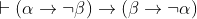b) Give a deduction or show that a deduction exists, i.e. you may use meta-rules such as hypothetical syllogism or the deduction theorem (but not completeness.)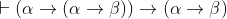We were unable to transcribe this image
We were unable to transcribe this image

#### Homework Answers

Answer #1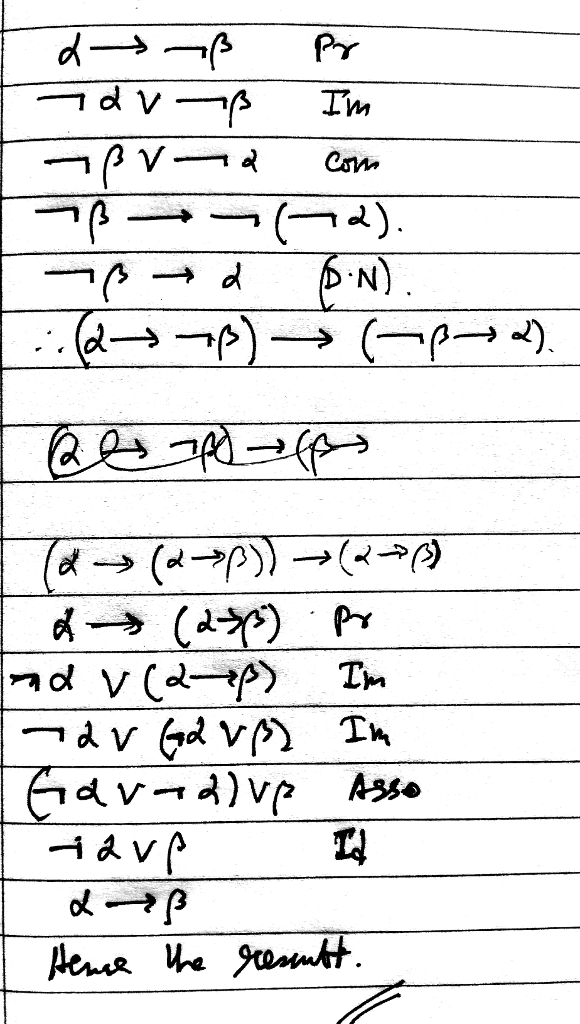Know the answer?
Your Answer:

#### Post as a guest

Your Name:

What's your source?

#### Earn Coin

Coins can be redeemed for fabulous gifts.

Not the answer you're looking for? Ask your own homework help question. Our experts will answer your question WITHIN MINUTES for Free.
Similar Homework Help Questions
• ### 45. Natural Deduction Practice 2 Aa Aa As you learn additional natural deduction rules, and as...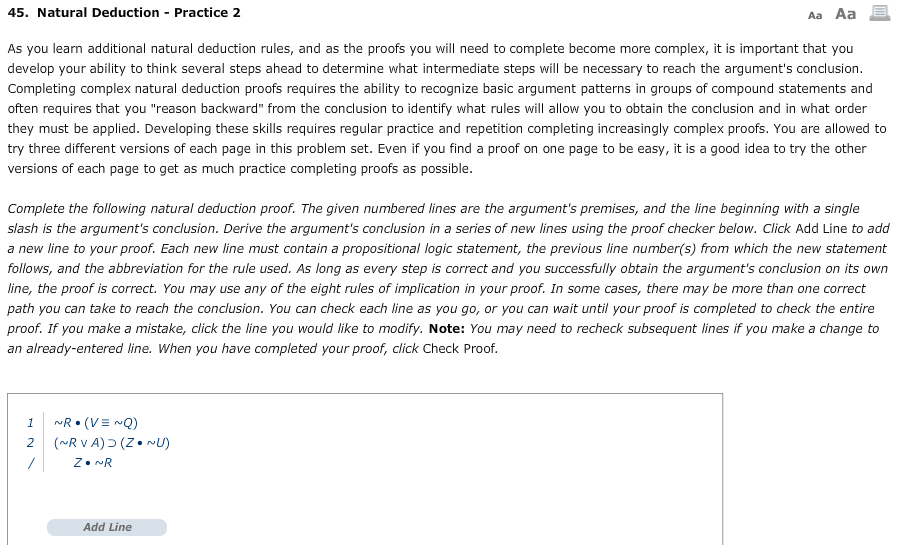45. Natural Deduction Practice 2 Aa Aa As you learn additional natural deduction rules, and as the proofs you will need to complete become more complex, it is important that you develop your ability to think several steps ahead to determine what intermediate steps will be necessary to reach the argument's conclusion. Completing complex natural deduction proofs requires the ability to recognize basic argument patterns in groups of compound statements and often requires that you "reason backward" from the conclusion...

• ### 13. Natural Deduction Practice 9 Aa Aa As you learn additional natural deduction rules, and as the proofs you will need to complete become more complex, it is important that you develop your ability...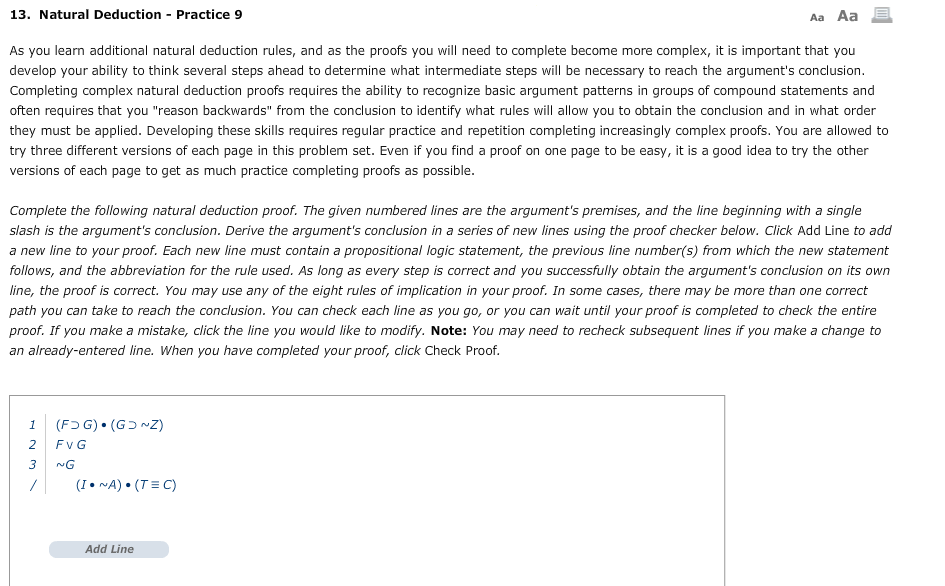13. Natural Deduction Practice 9 Aa Aa As you learn additional natural deduction rules, and as the proofs you will need to complete become more complex, it is important that you develop your ability to think several steps ahead to determine what intermediate steps will be necessary to reach the argument's conclusion Completing complex natural deduction proofs requires the ability to recognize basic argument patterns in groups of compound statements and often requires that you "reason backwards" from the conclusion...

• ### INSTRUCTIONS: Use natural deduction to derive the conclusion in each problem. Remember to number each additional line v...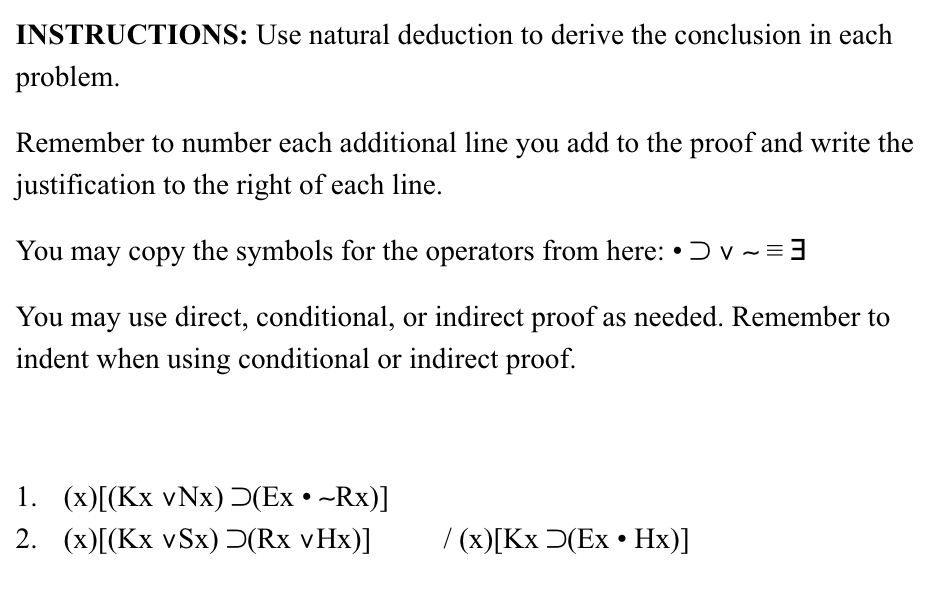INSTRUCTIONS: Use natural deduction to derive the conclusion in each problem. Remember to number each additional line vou add to the proof and write the justification to the right of each line. You may copy the symbols for the operators from here: . כ You may use direct, conditional, or indirect proof as needed. Remember to indent when using conditional or indirect proof. INSTRUCTIONS: Use natural deduction to derive the conclusion in each problem. Remember to number each additional line...

• ### INSTRUCTIONS: Use natural deduction to derive the conclusion in each problem. Remember to number each additional line y...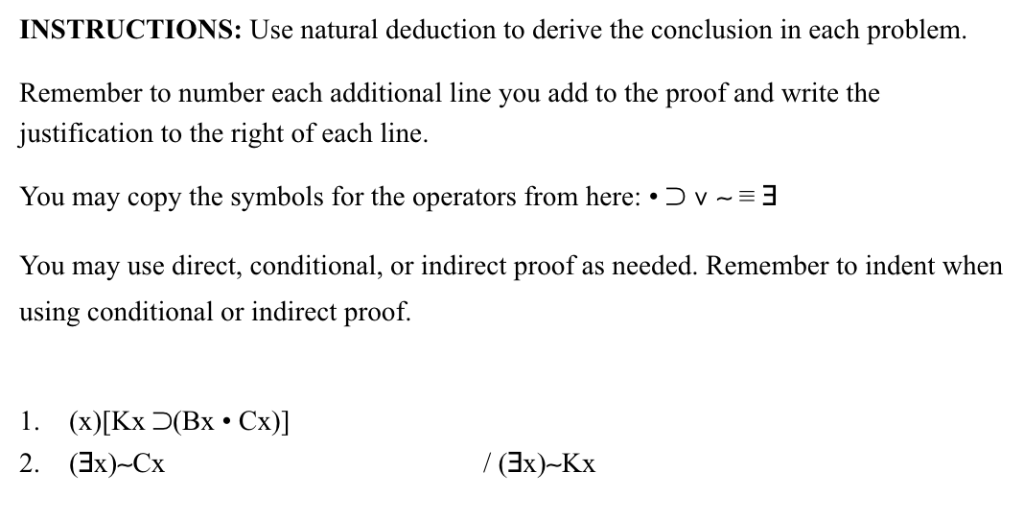INSTRUCTIONS: Use natural deduction to derive the conclusion in each problem. Remember to number each additional line you add to the proof and write the justification to the right of each line. You may copy the symbols for the operators from here: .Dv3 You may use direct, conditional, or indirect proof as needed. Remember to indent when using conditional or indirect proof. / (Bx)-Kx 2. (Bx)-Cx INSTRUCTIONS: Use natural deduction to derive the conclusion in each problem. Remember to number...

• ### INSTRUCTIONS: Use natural deduction to derive the conclusion in each problem. Remember to number each additional line y...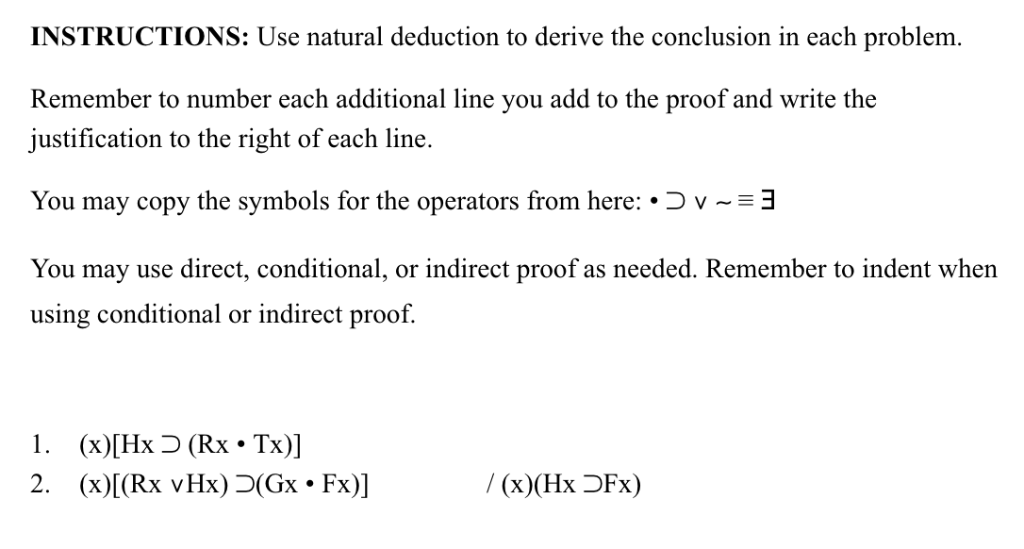INSTRUCTIONS: Use natural deduction to derive the conclusion in each problem. Remember to number each additional line you add to the proof and write the justification to the right of each line You may copy the symbols for the operators from here: .O v-3 You may use direct, conditional, or indirect proof as needed. Remember to indent when using conditional or indirect proof. 1. (x)[Hx D (Rx . Tx) / (x)(Hx OFx) INSTRUCTIONS: Use natural deduction to derive the conclusion...

• ### INSTRUCTIONS: Use natural deduction to derive the conclusion in each problem Remember to number each additional...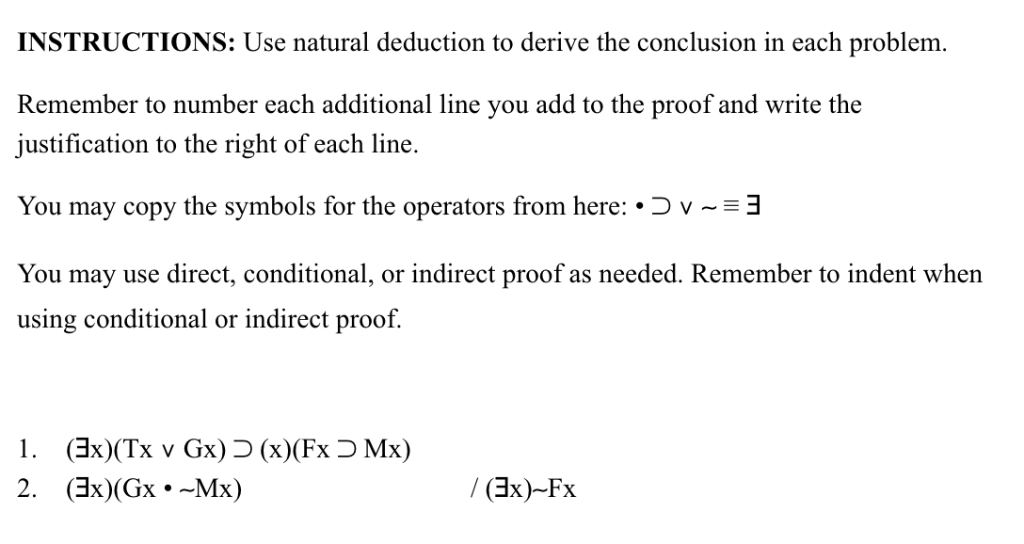INSTRUCTIONS: Use natural deduction to derive the conclusion in each problem Remember to number each additional line you add to the proof and write the ustification to the right of each line. You may copy the symbols for the operators from here: .O v-3 You may use direct, conditional, or indirect proof as needed. Remember to indent when using conditional or indirect proof. 2. (Bx)(Gx Mx) /(x)-Fx

• ### Instructions: For each of the following argument forms, complete a proof of validity, by natural deduction, USING ALL 19 RULES OF INFERENCE. Please note that some of the proofs may allow fo...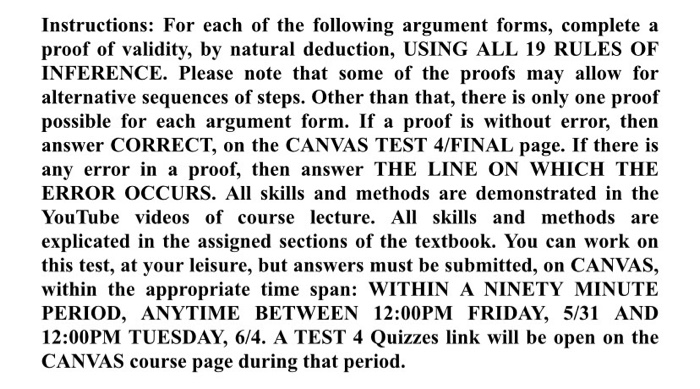Instructions: For each of the following argument forms, complete a proof of validity, by natural deduction, USING ALL 19 RULES OF INFERENCE. Please note that some of the proofs may allow for alternative sequences of steps. Other than that, there is only one proof possible for each argument form. If a proof is without error, then answer CORRECT, on the CANVAS TEST 4/FINAL page. If there is any error in a proof, then answer THE LINE ON WHICH THE ERROR...

• ### 1. Provide semi-formal Natural Deduction proofs of the following claims. You may only use the eight...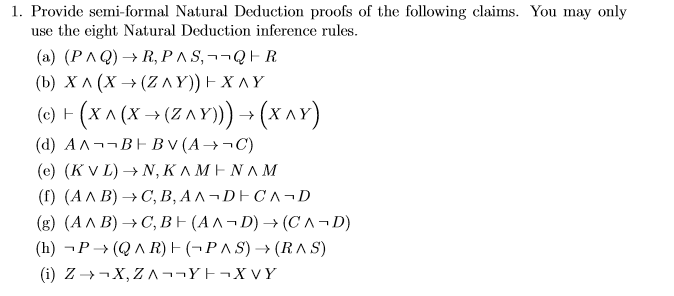1. Provide semi-formal Natural Deduction proofs of the following claims. You may only use the eight Natural Deduction inference rules. (a) (PAQ) + R,PAS,-QER (b) XA (X+(Z AY))-XAY (c) F(X A (X (ZAY)))(X AY) (d) AABEBV(A -C) (e) (KVL) +N, KAMENAM (f) (AAB) →C,B,AA-DECAD (g) (AAB) C,BF (AAD)+(CAD) (h) -P→ (QAR)F(PAS) → (RAS) (i) Z-X,ZAYE-XVY

• ### here is the dice images you need to use Problem 1/5 (20 points) Create a JavaFX...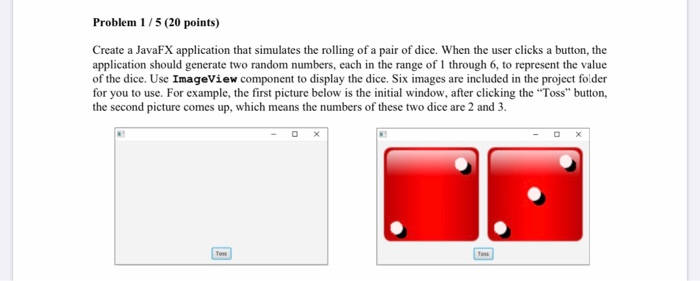here is the dice images you need to use Problem 1/5 (20 points) Create a JavaFX application that simulates the rolling of a pair of dice. When the user clicks a button, the application should generate two random numbers, each in the range of 1 through 6, to represent the value of the dice. Use ImageView component to display the dice. Six images are included in the project folder for you to use. For example, the first picture below is...

• ### You are given the following system of equations: Design a system which calculates x and y...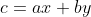You are given the following system of equations: Design a system which calculates x and y given a, b, c, d, e, and f. You may use any architecture of your choosing. You may also assume that n-bit dividers are available. c=ax + by We were unable to transcribe this image

Free Homework App

Scan Your Homework
to Get Instant Free Answers
Need Online Homework Help?

Get Answers For Free
Most questions answered within 3 hours.